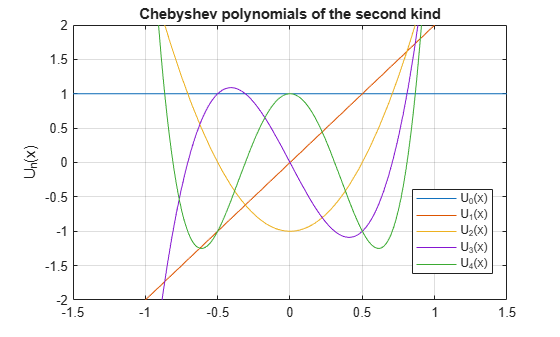# chebyshevU

Chebyshev polynomials of the second kind

## Syntax

``chebyshevU(n,x)``

## Description

example

````chebyshevU(n,x)` represents the `n`th degree Chebyshev polynomial of the second kind at the point `x`.```

## Examples

### First Five Chebyshev Polynomials of the Second Kind

Find the first five Chebyshev polynomials of the second kind for the variable `x`.

```syms x chebyshevU([0, 1, 2, 3, 4], x)```
```ans = [ 1, 2*x, 4*x^2 - 1, 8*x^3 - 4*x, 16*x^4 - 12*x^2 + 1]```

### Chebyshev Polynomials for Numeric and Symbolic Arguments

Depending on its arguments, `chebyshevU` returns floating-point or exact symbolic results.

Find the value of the fifth-degree Chebyshev polynomial of the second kind at these points. Because these numbers are not symbolic objects, `chebyshevU` returns floating-point results.

`chebyshevU(5, [1/6, 1/3, 1/2, 2/3, 4/5])`
```ans = 0.8560 0.9465 0.0000 -1.2675 -1.0982```

Find the value of the fifth-degree Chebyshev polynomial of the second kind for the same numbers converted to symbolic objects. For symbolic numbers, `chebyshevU` returns exact symbolic results.

`chebyshevU(5, sym([1/6, 1/4, 1/3, 1/2, 2/3, 4/5]))`
```ans = [ 208/243, 33/32, 230/243, 0, -308/243, -3432/3125]```

### Evaluate Chebyshev Polynomials with Floating-Point Numbers

Floating-point evaluation of Chebyshev polynomials by direct calls of `chebyshevU` is numerically stable. However, first computing the polynomial using a symbolic variable, and then substituting variable-precision values into this expression can be numerically unstable.

Find the value of the 500th-degree Chebyshev polynomial of the second kind at `1/3` and `vpa(1/3)`. Floating-point evaluation is numerically stable.

```chebyshevU(500, 1/3) chebyshevU(500, vpa(1/3))```
```ans = 0.8680 ans = 0.86797529488884242798157148968078```

Now, find the symbolic polynomial ```U500 = chebyshevU(500, x)```, and substitute `x = vpa(1/3)` into the result. This approach is numerically unstable.

```syms x U500 = chebyshevU(500, x); subs(U500, x, vpa(1/3))```
```ans = 63080680195950160912110845952.0```

Approximate the polynomial coefficients by using `vpa`, and then substitute `x = sym(1/3)` into the result. This approach is also numerically unstable.

`subs(vpa(U500), x, sym(1/3))`
```ans = -1878009301399851172833781612544.0```

### Plot Chebyshev Polynomials of the Second Kind

Plot the first five Chebyshev polynomials of the second kind.

```syms x y fplot(chebyshevU(0:4, x)) axis([-1.5 1.5 -2 2]) grid on ylabel('U_n(x)') legend('U_0(x)', 'U_1(x)', 'U_2(x)', 'U_3(x)', 'U_4(x)', 'Location', 'Best') title('Chebyshev polynomials of the second kind')```## Input Arguments

collapse all

Degree of the polynomial, specified as a nonnegative integer, symbolic variable, expression, or function, or as a vector or matrix of numbers, symbolic numbers, variables, expressions, or functions.

Evaluation point, specified as a number, symbolic number, variable, expression, or function, or as a vector or matrix of numbers, symbolic numbers, variables, expressions, or functions.

collapse all

### Chebyshev Polynomials of the Second Kind

• Chebyshev polynomials of the second kind are defined as follows:

`$U\left(n,x\right)=\frac{\mathrm{sin}\left(\left(n+1\right)a\mathrm{cos}\left(x\right)\right)}{\mathrm{sin}\left(a\mathrm{cos}\left(x\right)\right)}$`

These polynomials satisfy the recursion formula

`$U\left(0,x\right)=1,\text{ }U\left(1,x\right)=2\text{ }x,\text{ }U\left(n,x\right)=2\text{ }x\text{ }U\left(n-1,x\right)-U\left(n-2,x\right)$`
• Chebyshev polynomials of the second kind are orthogonal on the interval -1 ≤ x ≤ 1 with respect to the weight function $w\left(x\right)=\sqrt{1-{x}^{2}}$.

• Chebyshev polynomials of the second kind are a special case of the Jacobi polynomials

`$U\left(n,x\right)=\frac{{2}^{2n}n!\left(n+1\right)!}{\left(2n+1\right)!}P\left(n,\frac{1}{2},\frac{1}{2},x\right)$`

and Gegenbauer polynomials

`$U\left(n,x\right)=G\left(n,1,x\right)$`

## Tips

• `chebyshevU` returns floating-point results for numeric arguments that are not symbolic objects.

• `chebyshevU` acts element-wise on nonscalar inputs.

• At least one input argument must be a scalar or both arguments must be vectors or matrices of the same size. If one input argument is a scalar and the other one is a vector or a matrix, then `chebyshevU` expands the scalar into a vector or matrix of the same size as the other argument with all elements equal to that scalar.

 Hochstrasser, U. W. “Orthogonal Polynomials.” Handbook of Mathematical Functions with Formulas, Graphs, and Mathematical Tables. (M. Abramowitz and I. A. Stegun, eds.). New York: Dover, 1972.

#### Mathematical Modeling with Symbolic Math Toolbox

Get examples and videos